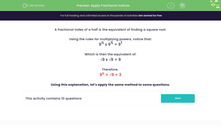# Apply Fractional Indices

In this worksheet, students will revise and apply fractional indices.Key stage:  KS 3

Curriculum topic:   Number

Curriculum subtopic:   Understand Integer Powers/Real Roots

Difficulty level:#### Worksheet Overview

A fractional index of a half is the equivalent of finding a square root.

Using the rules for multiplying powers, notice that:

9½ x 9½ = 91

Which is then the equivalent of:

√9 x √9 = 9

Therefore,

9½ = √9 = 3

Understanding this explanation is helpful, but the important thing is to remember that any number to the power of a half, simply means the square root of that number.

Shall we try some questions now?You can look back at this introduction if you need to by clicking on the red button at the side of the screen.

### What is EdPlace?

We're your National Curriculum aligned online education content provider helping each child succeed in English, maths and science from year 1 to GCSE. With an EdPlace account you’ll be able to track and measure progress, helping each child achieve their best. We build confidence and attainment by personalising each child’s learning at a level that suits them.

Get started